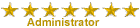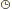DIỄN ĐÀN CỦA LỚP 10301 ĐH SPKT TP HCM Bạn có muốn phản ứng với tin nhắn này? Vui lòng đăng ký diễn đàn trong một vài cú nhấp chuột hoặc đăng nhập để tiếp tục.Welcome to our forum. We hope you can find something useful. For more information please contact nhuphuzz@yahoo.comForum hiện không còn được cập nhật. Những tài liệu cần thiết nếu các bạn cần có thể gửi mail yêu cầu cho ad, ad sẽ cố gắng giúp cho các bạn trong khả năng có thể. Email: nhuphuzz@yahoo.com
 DIỄN ĐÀN CỦA LỚP 10301 ĐH SPKT TP HCM :: HỌC HÀNH :: English Study

# How Can This Be True, Math Nerds?Tác giảThông điệp
nhuphuzzTổng số bài gửi : 432
Points : 14598
Reputation : 17
Join date : 18/10/2010Tiêu đề: How Can This Be True, Math Nerds?04/12/11, 02:53 pm How Can This Be True, Math Nerds?[You must be registered and logged in to see this image.]How Can This Be True - Answer1. For both of the pictures to be exactly the same, they must bothhave the same area AND each of the pictures must have same area as thearea of the "PERFECT" triangle (oh no - shades of dreaded Math come to mind!) ...Here are the calculated areas 4U:Hint: the area of a triangle = (1/2)b.hwhere b is the base and h is the height1A. Before looking at either picture, calculate what the area of this picturewould be if it was a PERFECT triangle (i.e. 13 X 5 squares)Area = (1/2)b.h = (1/2)(13)(5) = 65/2 = 32 + 1/21B. Area of the top pictureArea = 1 + 2 + 3 + 4= (1/2)(5)(2) + 1/2(8)(3) + 7 + 8= 5 + 12 + 7 + 8 = 321C. Area of the lower pictureArea = 1 + 2 + 3 + 4 + 5= (1/2)(5)(2) + (1/2)(8)(3) + 7 + 8 + 1= 5 + 12 + 7 + 8 + 1 = 33Neither of the two pictures form a perfect triangle,but both of them look very close to the outline of a perfect triangle! 2. Compare the slopes of the triangles and you realize thatthe hypotenuse of the overall triangle is not a straight line ...Hint: the slope of a line = rise / run(the slope of a horizontal line is zero)2A. Slope of the PERFECT triangleSlope = rise / run = 5 / 13 = 0.3846152B. Slope of Triangle 1 (in both pictures)Slope = rise / run = 2 / 5 = 0.42C. Slope of Triangle 2 (in both pictures)Slope = rise / run = 3 / 8 = 0.375Prove it by blowing up the images and running a ruler along thehypotenuse of both triangles - both are not a perfect line (but close!) 3. The bottom triangle has ~181.2 degrees when by definition a triangle must have only 180 degrees. I used the inverse tangent function and applied it to both angles that were not right angles. I calculated both what the angles should be based on the lengths of the whole triangle at the bottom and what they actually were based on the smaller triangles that formed the angles. The top right angle of the bottom triangle should be ~68.96 degrees based on tan^-1(13/5) but when you input the lengths of the smaller angle into this formula (tan^-1(8/3)) the angle comes out to be 69.44 degrees. Likewise with the other angle it should be ~21.04 degrees and actually is ~21.8. Larger angles mean more area into the shape. Conclusion: the 2 objects are NOT triangles(even though they at first appear so!)and they have different areas... Thanks to Paul Stafford, Eric Nelson, Roy Bishop & freeclimber!Được sửa bởi nhuphuzz ngày 04/12/11, 02:59 pm; sửa lần 1.nhuphuzzTổng số bài gửi : 432
Points : 14598
Reputation : 17
Join date : 18/10/2010Tiêu đề: Re: How Can This Be True, Math Nerds?04/12/11, 02:55 pm This answer is very clear, right?How Can This Be True, Math Nerds?Trang 1 trong tổng số 1 trang

Permissions in this forum:Bạn không có quyền trả lời bài viếtChuyển đến: Chọn Diễn Đàn||---------Thông Báo-------|--Hướng dẫn cách đăng bài|--Diễn Đàn|   |--Thông tin liên lạc|   |--Ảnh lưu niệm|   |--Phần mềm và hướng dẫn sử dụng|   |--trao đổi ý kiến và bày tỏ cảm xúc|   |--mua bán|   |--Tin Tức|   |--Đăng Kí Môn Học|   |--Vi Điều Khiển|   |--Góp Ý|   |--HỌC HÀNH    |--Học Kỳ I    |   |--Nhập môn logic học    |   |--Xác suất thống kê    |   |--Toán cao cấp A3    |   |--Pháp luật đại cương    |   |--Anh văn    |   |--Ngôn ngữ lập trình    |   |--Điện tử cơ bản    |   |--Mạch điện    |   |--Nguồn điện đặc biệt    |   |--Hệ thống điều khiển tự động    |   |--Đồ án môn học 1    |       |--Học Kỳ II    |   |--Toán cao cấp A4    |   |--Hàm biến phức và phép biến đổi Laplace    |   |--Anh văn chuyên ngành    |   |--Vật lý đại cương A3    |   |--Chuyên đề công nghệ mới    |   |--Điều khiển lập trình nâng cao    |   |--Vi xử lý    |   |--Đồ án môn học 2    |   |--Kỹ thuật PLD và ASIC    |   |--Lý thuyết tín hiệu    |   |--Hệ thống thu thập dữ liệu và điều khiển    |   |--Giáo dục quốc phòng    |       |--Học kỳ III    |   |--TT PLD và ASIC    |   |--TT điểu khiển lập trình nâng cao    |   |--Chuyên đề hệ thống cơ điện tử    |   |--Độ tin cậy của hệ thống    |   |--Điều khiển thông minh    |   |--Công nghệ RFID    |   |--Kỹ thuật siêu âm    |   |--Quản lý công nghiệp    |   |--Công nghệ nano    |       |--English Study        |--Word of The Day